Publicité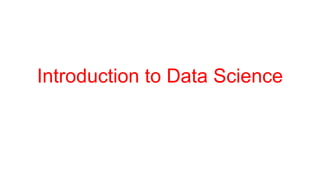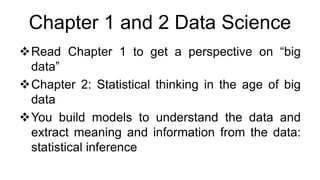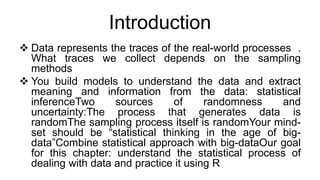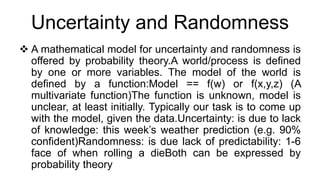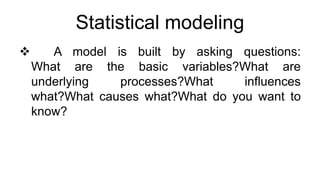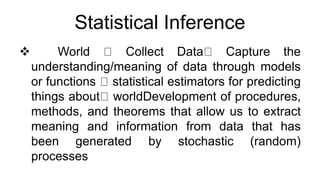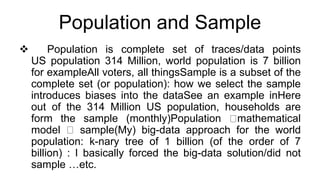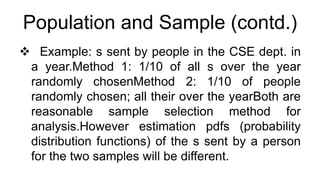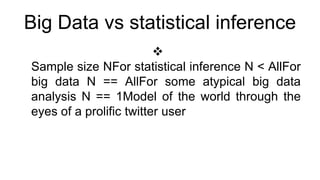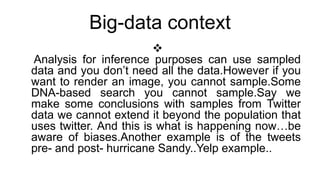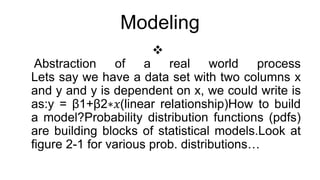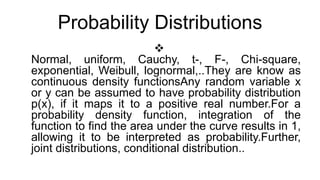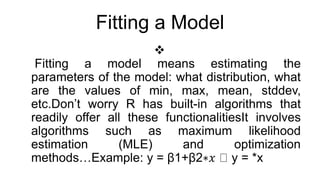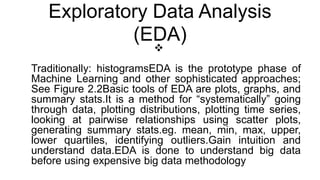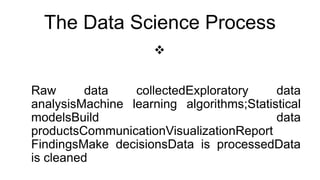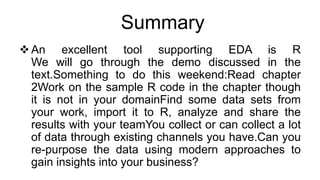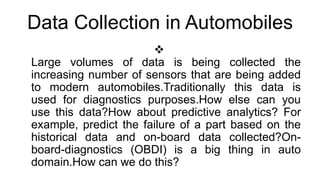1 sur 17
Publicité

### UNIT1-2.pptx

1. Introduction to Data Science
2. Chapter 1 and 2 Data Science Read Chapter 1 to get a perspective on “big data” Chapter 2: Statistical thinking in the age of big data You build models to understand the data and extract meaning and information from the data: statistical inference
3. Introduction  Data represents the traces of the real-world processes . What traces we collect depends on the sampling methods  You build models to understand the data and extract meaning and information from the data: statistical inferenceTwo sources of randomness and uncertainty:The process that generates data is randomThe sampling process itself is randomYour mind- set should be “statistical thinking in the age of big- data”Combine statistical approach with big-dataOur goal for this chapter: understand the statistical process of dealing with data and practice it using R
4. Uncertainty and Randomness  A mathematical model for uncertainty and randomness is offered by probability theory.A world/process is defined by one or more variables. The model of the world is defined by a function:Model == f(w) or f(x,y,z) (A multivariate function)The function is unknown, model is unclear, at least initially. Typically our task is to come up with the model, given the data.Uncertainty: is due to lack of knowledge: this week’s weather prediction (e.g. 90% confident)Randomness: is due lack of predictability: 1-6 face of when rolling a dieBoth can be expressed by probability theory
5. Statistical modeling  A model is built by asking questions: What are the basic variables?What are underlying processes?What influences what?What causes what?What do you want to know?
6. Statistical Inference  World Collect Data Capture the understanding/meaning of data through models or functions statistical estimators for predicting things about worldDevelopment of procedures, methods, and theorems that allow us to extract meaning and information from data that has been generated by stochastic (random) processes
7. Population and Sample  Population is complete set of traces/data points US population 314 Million, world population is 7 billion for exampleAll voters, all thingsSample is a subset of the complete set (or population): how we select the sample introduces biases into the dataSee an example inHere out of the 314 Million US population, households are form the sample (monthly)Population mathematical model sample(My) big-data approach for the world population: k-nary tree of 1 billion (of the order of 7 billion) : I basically forced the big-data solution/did not sample …etc.
8. Population and Sample (contd.)  Example: s sent by people in the CSE dept. in a year.Method 1: 1/10 of all s over the year randomly chosenMethod 2: 1/10 of people randomly chosen; all their over the yearBoth are reasonable sample selection method for analysis.However estimation pdfs (probability distribution functions) of the s sent by a person for the two samples will be different.
9. Big Data vs statistical inference  Sample size NFor statistical inference N < AllFor big data N == AllFor some atypical big data analysis N == 1Model of the world through the eyes of a prolific twitter user
10. Big-data context  Analysis for inference purposes can use sampled data and you don’t need all the data.However if you want to render an image, you cannot sample.Some DNA-based search you cannot sample.Say we make some conclusions with samples from Twitter data we cannot extend it beyond the population that uses twitter. And this is what is happening now…be aware of biases.Another example is of the tweets pre- and post- hurricane Sandy..Yelp example..
11. Modeling  Abstraction of a real world process Lets say we have a data set with two columns x and y and y is dependent on x, we could write is as:y = β1+β2∗𝑥(linear relationship)How to build a model?Probability distribution functions (pdfs) are building blocks of statistical models.Look at figure 2-1 for various prob. distributions…
12. Probability Distributions  Normal, uniform, Cauchy, t-, F-, Chi-square, exponential, Weibull, lognormal,..They are know as continuous density functionsAny random variable x or y can be assumed to have probability distribution p(x), if it maps it to a positive real number.For a probability density function, integration of the function to find the area under the curve results in 1, allowing it to be interpreted as probability.Further, joint distributions, conditional distribution..
13. Fitting a Model  Fitting a model means estimating the parameters of the model: what distribution, what are the values of min, max, mean, stddev, etc.Don’t worry R has built-in algorithms that readily offer all these functionalitiesIt involves algorithms such as maximum likelihood estimation (MLE) and optimization methods…Example: y = β1+β2∗𝑥 y = *x
14. Exploratory Data Analysis (EDA)  Traditionally: histogramsEDA is the prototype phase of Machine Learning and other sophisticated approaches; See Figure 2.2Basic tools of EDA are plots, graphs, and summary stats.It is a method for “systematically” going through data, plotting distributions, plotting time series, looking at pairwise relationships using scatter plots, generating summary stats.eg. mean, min, max, upper, lower quartiles, identifying outliers.Gain intuition and understand data.EDA is done to understand big data before using expensive big data methodology
15. The Data Science Process  Raw data collectedExploratory data analysisMachine learning algorithms;Statistical modelsBuild data productsCommunicationVisualizationReport FindingsMake decisionsData is processedData is cleaned
16. Summary An excellent tool supporting EDA is R We will go through the demo discussed in the text.Something to do this weekend:Read chapter 2Work on the sample R code in the chapter though it is not in your domainFind some data sets from your work, import it to R, analyze and share the results with your teamYou collect or can collect a lot of data through existing channels you have.Can you re-purpose the data using modern approaches to gain insights into your business?
17. Data Collection in Automobiles  Large volumes of data is being collected the increasing number of sensors that are being added to modern automobiles.Traditionally this data is used for diagnostics purposes.How else can you use this data?How about predictive analytics? For example, predict the failure of a part based on the historical data and on-board data collected?On- board-diagnostics (OBDI) is a big thing in auto domain.How can we do this?
Publicité